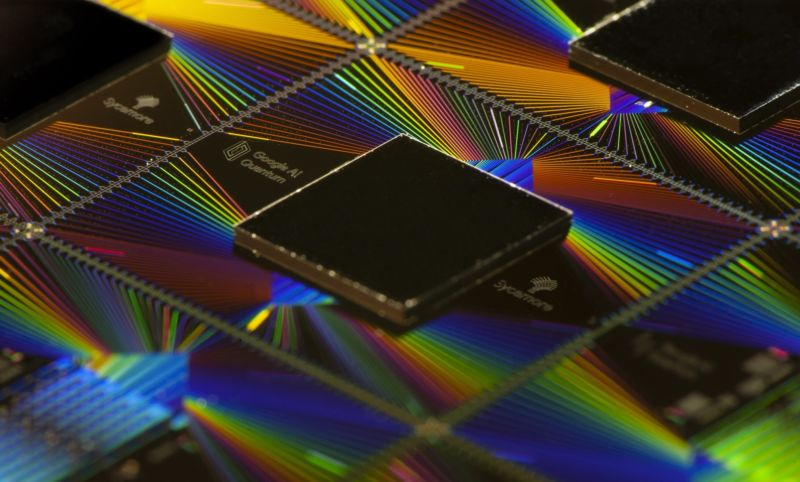# Quantum computer succeeds where a classical algorithm fails

People have performed many mathematical proofs to show that a quantum computer will vastly outperform traditional computers on a number of algorithms. But the quantum computers we have now are error-prone and don’t have enough qubits to allow for error correction. The only demonstrations we’ve had involve quantum computing hardware evolving out of a random configuration and traditional computers failing to simulate their normal behavior. Useful calculations are an exercise for the future.

But a new paper from Google’s quantum computing group has now moved beyond these sorts of demonstrations and used a quantum computer as part of a system that can help us understand quantum systems in general, rather than the quantum computer. And they show that, even on today’s error-prone hardware, the system can outperform classical computers on the same problem.

## Probing quantum systems

To understand what the new work involves, it helps to step back and think about how we typically understand quantum systems. Since the behavior of these systems is probabilistic, we typically need to measure them repeatedly. The results of these measurements are then imported into a classical computer, which processes them to generate a statistical understanding of the system’s behavior. With a quantum computer, by contrast, it can be possible to mirror a quantum state using the qubits themselves, reproduce it as often as needed, and manipulate it as necessary. This method has the potential to provide a route to a more direct understanding of the quantum system at issue.

Much of the paper is devoted to describing situations where this should be the case, in part elaborating on ideas described in earlier papers.

The first of these ideas describes some property of a quantum system involving an arbitrary number of items—like a quantum computer with n qubits. This is exactly the circumstance described above, where repeated measurements need to be made before a classical computer can reliably identify a property. By contrast, a quantum computer can store a copy of the system in its memory, allowing it to be repeatedly duplicated and processed.

These problems, the authors show, can be solved on a quantum computer in what’s called polynomial time, where the number of qubits is raised to a constant power (denoted nk). Using classical hardware, by contrast, the time scales as a constant raised to the power related to the number of qubits. As the number of qubits increases, the time needed for classical hardware rises much faster.

## Options two and three

The second task they identify is a quantum principal component analysis, where computers are used to identify the property that has the largest influence on the quantum system’s behavior. This was chosen in part because this analysis is thought to be relatively insensitive to the noise introduced by errors in today’s quantum processors. Mathematically, the team shows that the number of times you’d need to repeat the measurements for analysis on a classical system grows exponentially with the number of qubits. Using a quantum system, the analysis can be done with a constant number of repeats.

The final situation involves allowing a physical process to influence the state of a quantum system, causing it to evolve to a new state. The goal is to find a model of the process that can accurately predict what the new state would be. Again, using a classical system means the challenge of getting enough measurements scales exponentially with the number of qubits but grows much more slowly when quantum computing is used.

Why does a quantum computer perform so much better? The researchers say that a key step is storing two copies of the examined system and then entangling them. This method is something that’s only possible on quantum hardware.

https://arstechnica.com/science/2022/06/quantum-computer-succeeds-where-a-classical-algorithm-fails/Previous post China Is Still Busy Hacking Into Carrier Networks to Spy on Users, US WarnsNext post Deals Reminder: LutiBand Smartwatch, Save 41%